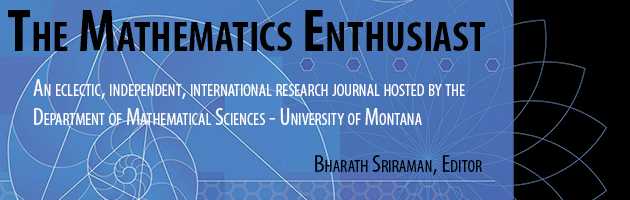•
•13

3

#### Abstract

The decimal expansions of the numbers 1/n (such as 1/3 = .03333..., 1/7 = 0.142857...) are most often viewed as tools for approximating quantities to a desired degree of accuracy. The aim of this exposition is to show how these modest expressions in fact have much more to offer, particularly in the case when the expansions are infinitely long. First we discuss how simply asking about the period (that is, the length of the repeating sequence of digits) of the decimal expansion of 1/n naturally leads to more sophisticated ideas from elementary number theory, as well as to unsolved mathematical problems. Then we describe a surprising theorem of K. Girstmair showing that the digits of the decimal expansion of 1/p, for certain primes p, secretly contain deep facts that have long delighted algebraic number theorists.

189

216

COinS